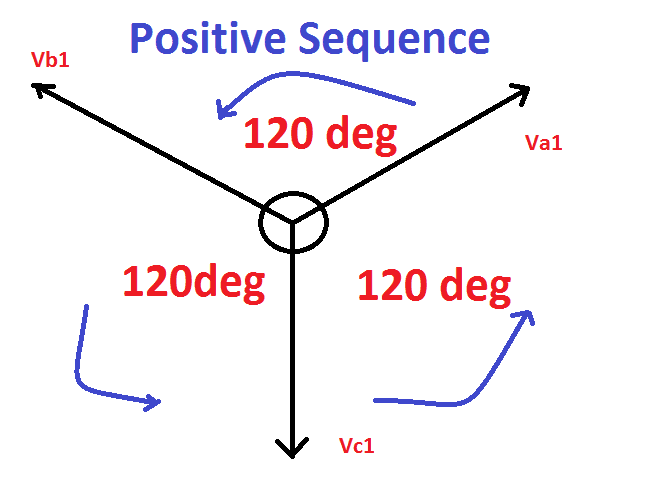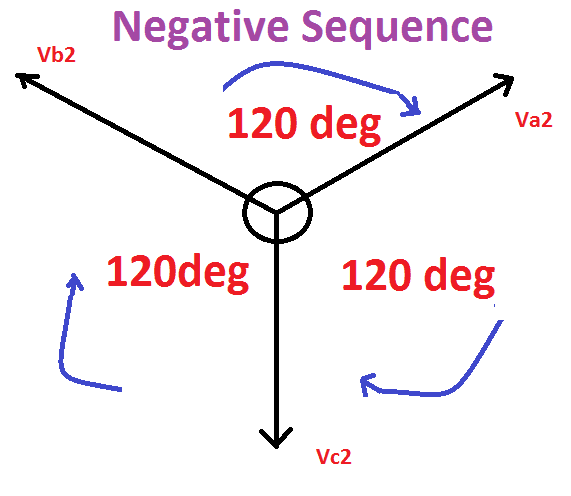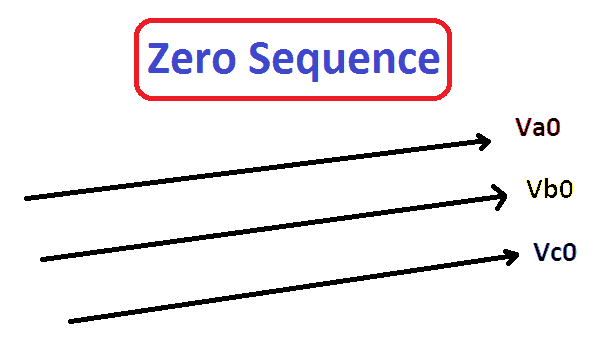# What is Sequence Impedance

## What is Sequence Impedance:

The sequence impedance of the circuit tells about the behavior of the system during any type of asymmetrical fault condition i.e line to line fault or line to ground fault or line to line to line to ground (L-L-L-G) fault. The entire performance of the power system can be calculated by the impedance offered by the different element of the power system to the flow of the different phase sequence component of the current (flow of electron). The Sequence Impedance is generally used to simplifies the asymmetrical fault calculations.  The sequence impedance of a power system consists of Positive sequence impedance, negative sequence impedance and zero sequence impedance. Let see what are they…

### Positive Sequence Impedance:

The impedance offered by the flow of positive sequence current in the circuit is called the positive sequence impedance. Here the positive sequence means all the electrical quantities are having equal magnitude and displaced 120deg each other.Positive sequence Impedance is denoted by Z1

### Negative Sequence Impedance:

The impedance offered by the flow of negative sequence current in the circuit is called the negative sequence impedance. Here the negative sequence indicates all the electrical quantities are having equal magnitude and displaced 120deg each other in opposite direction.Negative Sequence impedance is denoted by Z2

### Zero Sequence Impedance:

The impedance offered by the flow of Zero sequence current in the circuit is called the zero sequence impedance. Here the positive sequence means all the electrical quantities are having equal magnitude and all are in phase.Zero Sequence impedance is denoted by Z

The sequence impedance varies with the type of power system components under consideration: –

The positive sequence impedance and negative sequence impedance for transformers and power lines are same Since both are static and balanced power system components. But in case of rotating machines the positive and negative sequence impedance are different because of impedance of the winding is depending on the magnetic circuits and the MOC of the winding.